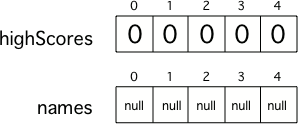# 6.5. Unit 6 Summary¶

In this chapter you learned about Arrays. An array is consecutive storage for multiple items of the same type like the top five scores in a game. You learned how to declare arrays, create them, and access array elements. Array elements are accessed using an index. The first element in an array is at index 0.Figure 1: Two 5 element arrays with their values set to the default values for integer and object arrays.¶

## 6.5.1. Concept Summary¶

• Array - An array can hold many items (elements) of the same type. You can access an item (element) at an index and set an item (element) at an index.

• Array Declaration - To declare an array specify the type of elements that will be stored in the array, then ([]) to show that it is an array of that type, then at least one space, and then a name for the array. Examples: int[] highScores; String[] names;

• Array Creation - To create an array type the name and an equals sign then use the new keyword, followed by a space, then the type, and then in square brackets the size of the array (the number of elements it can hold). Example: names = new String;

• Array Index - You can access and set values in an array using an index. The first element in an array called arr is at index 0 arr. The last element in an array is at the length minus one - arr[arr.length - 1].

• Array Initialization - You can also initialize (set) the values in the array when you create it. In this case you don’t need to specify the size of the array, it will be determined from the number of values that you specify. Example: int[] highScores = {99,98,98,88,68};

• Array Length - The length of an array is the number of elements it can hold. Use the public length field to get the length of the array. Example: given int[] scores = {1,2,2,1,3,1};, scores.length equals 6.

• Element Reference - A specific element can be referenced by using the name of the array and the element’s index in square brackets. Example: scores will return the 4th element (since index starts at 0, not 1). To reference the last element in an array, use array[array.length - 1]

• For-each Loop - Used to loop through all elements of an array. Each time through the loop the loop variable will be the next element in the array starting with the element at index 0, then index 1, then index 2, etc.

• Out of Bounds Exception - An error that means that you tried to access an element of the array that doesn’t exist maybe by doing arr[arr.length]. The first valid indices is 0 and the last is the length minus one.

## 6.5.2. Java Keyword Summary¶

• for - starts both a general for loop and a for-each loop. The syntax for a for each loop is for (type variable : array). Each time through the loop the variable will take on the next value in the array. The first time through the loop it will hold the value at index 0, then the value at index 1, then the value at index 2, etc.

• static - used to create a class method, which is a method that can be called using the class name like Math.abs(-3).

## 6.5.3. Vocabulary Practice¶

For more practice, see this Quizlet.

## 6.5.4. Common Mistakes¶

• forgetting to create the array - only declaring it (int[ ] nums;)

• using 1 as the first index not 0

• using array.length as the last valid index in an array, not array.length - 1.

• using array.length() instead of array.length (not penalized on the free response)

• using array.get(0) instead of array (not penalized on the free response)

• going out of bounds when looping through an array (using index <= array.length). You will get an ArrayIndexOutOfBoundsException.

• jumping out an loop too early by using one or more return statements before every value has been processed.